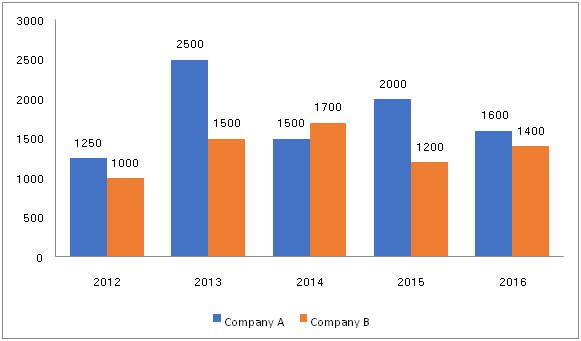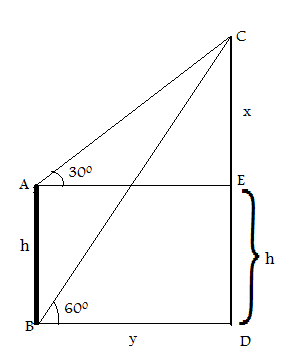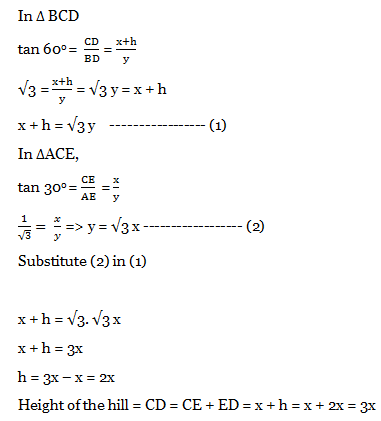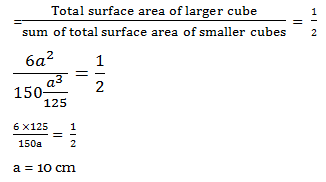# SSC CGL EXAMS 2018 | Quantitative Aptitude Practice Questions (Day-15)

Dear Aspirants, Here we have given the Important SSC Exam 2018 Practice Test Papers. Candidates those who are preparing for SSC 2018 can practice these questions to get more confidence to Crack SSC 2018 Examination.

[WpProQuiz 4644]

1) Find the value of sin2 7 ½° + sin2 82 ½° + tan2 2° . tan2 88° is

a) 2

b) 1

c) 0

d) 4

2) The angle of elevation of the summit of a hill the top and bottom of a nearby pole are 300 and 600 respectively. If the height of the pole is h, find the height of the hill.

a) 2h

b) √3h

c) √3x

d) 3x

3) A larger cube of certain side is divided into smaller cubes of side 5 cm. If the ratio of the surface area of larger cube to the sum of the surface area of the smaller cubes 1: 2. Find the length of side of the larger cube.

a) 10 cm

b) 20 cm

c) 15 cm

d) 12 cm

4) Find the value of 1 – 2 sin2 θ + sin4 θ.

a) 1 – sin4 θ

b) sin2 θ. Cos2 θ

c) cos4 θ

d) sin4 θ

5) Amar is 3 times faster than suman. Suman can complete a work in 15 days. If they work together then how long the work will complete?

a) 4 days

b) 3 ½ days

c) 3 ¾ days

d) 4 ½ days

6) A certain amount of money triples itself in 5 years at simple interest per annum. Find the rate of interest of the amount?

a) 10%

b) 20 %

c) 40%

d) 60%

7) If x = 6 + 26, then what is the value of √(x-1)+ 1/√(x – 1)

a) 5√3

b) 2√3

c) 3√2

d) 5√2

Direction (8 – 10): Read the instruction carefully and answer the questions.

Given line graph indicates the employee details of two companies.  Total employees are working in various years of company A and company B is given below8) If in Company A, 45% of employees are females in 2015 and 2016 respectively. Then find the ratio of total males of company A in the same years?

a) 2: 5

b) 5: 4

c) 3: 5

d) 5: 2

9) What is the average of total workers of Company B  between 2012 and 2015?

a) 1250

b) 1300

c) 1350

d) 1400

10) If in the year 2017, number of employees in company A and B were increased 30% and 40% respectively with respect to previous year. Then the total employees worked in the both the companies in 2017 are?

a) 4004

b) 4040

c) 4440

d) 4044

sin2 7 ½° + sin2 82 ½° + tan2 2° + tan2 88°

Sin 7 ½° = cos 82 ½ °

Similarly, tan2 88 = cos2

(cos 82 ½ °+ sin2 82 ½° )+ (tan2 2°. Cot22°) = 1 + 1 = 2 [∵ sin2 θ + cos2 θ = 1]

∵ [Cot22° = 1/ tan22°]Let AB is height of the pole = h

Let CD is the height of the hill = x + h

Let distance between the pole and hill = BD = y

CE = xSide of the larger cube =a

Volume of the larger cube = a3

Side of smaller cube = 5 cm

Volume of the smaller cube = 53 = 125 cm

Total surface area of larger cube = 6a2

Total surface area of smaller cubes = 6 (5)2 = 6 * 25 = 150 cm2

Sum of total surface area of smaller cubes = 150 * (a3/125)

[∵Total surface area of smaller cubes*(volume of the larger cube/ volume of the smaller cube)]1 – 2 sin2 θ + sin4 θ

= 12 – 2(1) (sin2θ) + sin4 θ = (1 – sin2θ)2 [∵ (a – b) = a2 + b2 – 2ab]

(1 – sin2θ)2 = (sin2 θ + cos2 θ – sin2θ) 2  [∵Sin2 θ + cos2 θ = 1  ]

= (cos2 θ)2 = cos4 θ

Work ratio of Amar and Suman = 3 : 1

Time = 1 : 3

Amar = 1/ 15

Suman = 3 /1 5= 5 days=(3/15)*5

1/15 + 1/5 = (1+ 3) / 15

The work will complete = 15 / 4 = 3 ¾ days

If the sum of money becomes n times in the n years at simple interest, then the rate of interest will be

R = 100 (multiply times – 1)/no of years

Rate = 100 (3 – 1)/5 = 100 *2/5 = 40%

x-1 = 5 + 2√6.

Then,

x-1 = 3 + 2 + 2√((3)(2))

∴ x-1 = (√3)² + (√2)² + 2(√3)(√2) = (√3 + √2)²

∴ √x-1 = √3 + √2

∴ 1/(√x-1) = 1/(√3 + √2) = √3 – √2

∴ (√x-1) + (1/√x-1) = (√3 + √2) + (√3 – √2)

= 2√3

Direction (8-10) :

Total Employees in 2015 in company A = 2000

Total Males in 2015 = 2000 * (55/100) = 20* 55

Total Employees in 2016 in company A = 1600

Total Males in 2016 = 1600 * (55/100) = 16* 55

Ratio of Males 2015 : male 2016

= 20* 55 : 16 : 55

= 5 : 4

Sum of the workers 2012 to 2015 of company B = 1000 + 1500 + 1700 + 1200

= 5400/4 = 1350

Number of employees in companies A in 2016 = 1600

Number of employees in companies A in 2017 = 1600(130/100) = 2080

Number of employees in companies B in 2016 = 1400

Number of employees in companies B in 2017 = 1400 (140/100) = 1960

Sum of employees in 2017 = 2080 + 1960 = 4040

### *******************